# Math in Focus Grade 3 Chapter 7 Practice 2 Answer Key Multiplying Without Regrouping

Go through the Math in Focus Grade 3 Workbook Answer Key Chapter 7 Practice 2 Multiplying Without Regrouping to finish your assignments.

## Math in Focus Grade 3 Chapter 7 Practice 2 Answer Key Multiplying Without Regrouping

Fill in the missing numbers.

Example
2 × 13 = ?
2 × 3 ones = 6 ones.
2 × 1 ones = 2 tens.
so, 2 × 13 = 26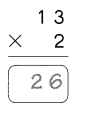Question 1.
2 × 342 = ?
2 × ___ ones = ___ ones
2 × ___ tens = ___ tens
2 × ___ hundreds = ___ hundreds
So, 2 × 342 = ___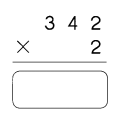2 × 342 = 684.

Explanation:
Given to find 2 × 342, so in the first step, we will multiply
2 × 2 ones = 4 ones, and then we will multiply
2 × 4 tens = 8 tens, and then we will multiply
2 × 3 hundreds = 6 hundreds
So, 2 × 342 = 684.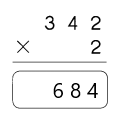Question 2.
3 × 312 = ?
3 × ___ ones = ___ ones
3 × ___ tens = ___ tens
3 × ___ hundreds = ___ hundreds
So, 3 × 312 = ___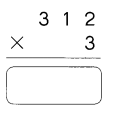3 × 312 = 936.

Explanation:
Given that to find 2 × 342, so in the first step, we will multiply
3 × 2 ones = 6 ones, and then we will multiply
3 × 1 tens = 3 tens, and then we will multiply
3 × 3 hundreds = 9 hundreds
So, 3 × 312 = 936.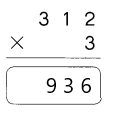Question 3.
4 × 201 = ?
4 × ___ one = ___ ones
3 × ___ tens = ___ tens
4 × ___ hundreds = ___ hundreds
So, 4 × 201 = ___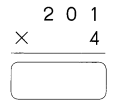4 × 201 = 804.

Explanation:
Given that 4 × 201, so in the first step, we will multiply
4 × 1 one = 4 ones, and then we will multiply
4 × 0 tens = 0 tens, and then we will multiply
4 × 2 hundreds = 8 hundreds
So, 4 × 201 = 804.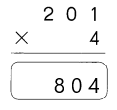Multiply.

Example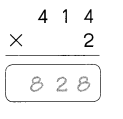Question 4.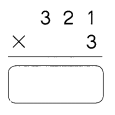321 × 3 = 963.

Explanation:
Here, the multiplication of 321 × 3 is 963.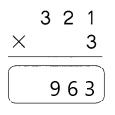Question 5.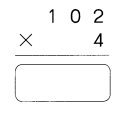102 × 4 = 408.

Explanation:
Here, the multiplication of 102 × 4 is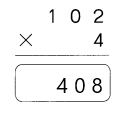Question 6.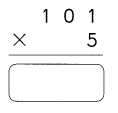101 × 5 = 505.

Explanation:
Here, the multiplication of 101 × 5 is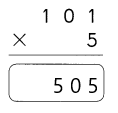Match each caterpillar to its leafs.

Question 7.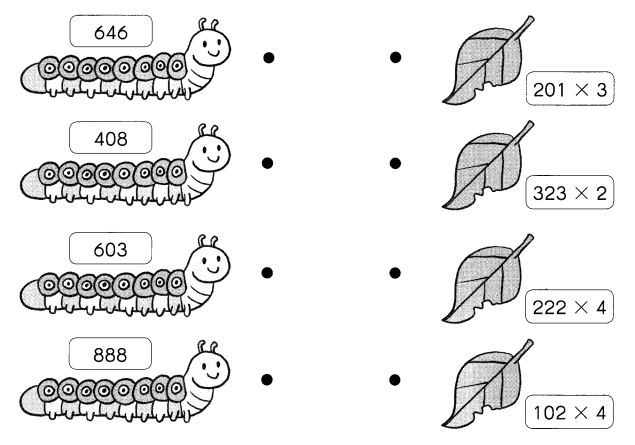Here, we have matched the product and the expression.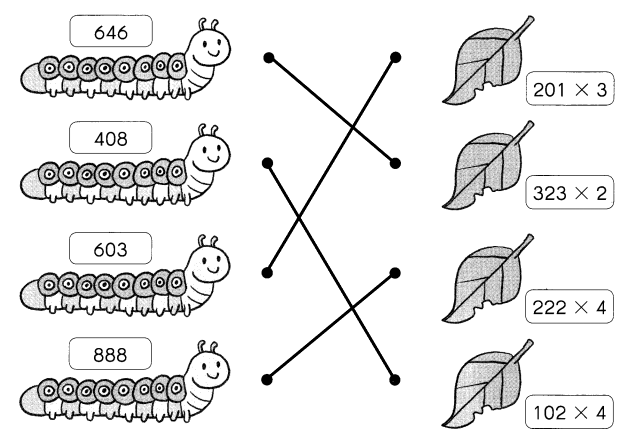Multiply and complete.

Question 8.
Frederick Frog is hungry. He is out hunting for his lunch. Clever Frederick Frog eats only non-poisonous flies. Flies carrying a product greater than 400 are non-poisonous. Which flies should Frederick Frog eat?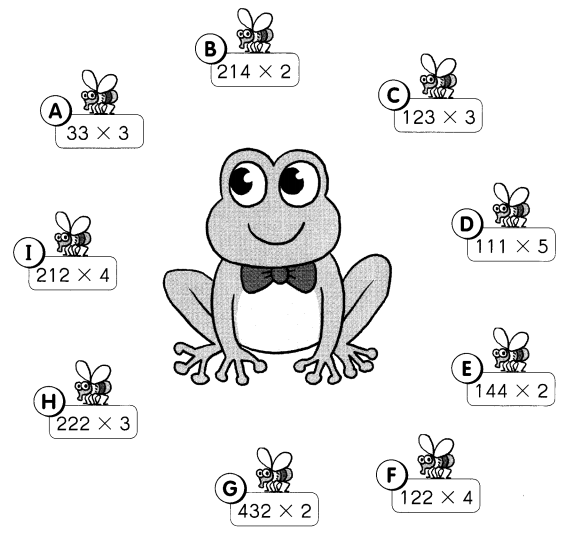Frederick Frog Should eat flies _____
Frederick Frog Should eat flies is B, D, F, G, H, I.

Explanation:
Given that Frederick Frog eats only non-poisonous flies, as the frog only eats non-poisonous which carries a product greater than 400. So the product which is greater than 400 is
432 × 2 is 864,
122 × 4 is 488,
222 × 3 is 666,
111 ×  5 is 555,
214 × 2 is 428,
212 × 2 is 424.
So Frederick Frog Should eat flies is B, D, F, G, H, I.

Solve.

Example
Curtis scores 22 points in a game.
He wants to score the same number of points for every game. How many points does he hope to score in 4 games?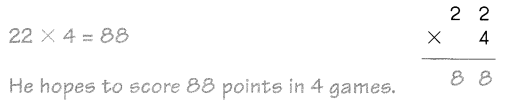The number of points does he hope to score in 4 games is 88 points.

Explanation:
Given that Curtis scores 22 points in a game and he wants to score the same number of points for every game. So the number of points does he hope to score in 4 games is 22 × 4 which is 88 points.

Question 9.
143 people ride the train every hour. How many people ride the train in 2 hours?
The number of people riding the train in 2 hours is 268 people.

Explanation:
Given that 143 people ride the train every hour, so the number of people riding the train in 2 hours is 143 × 2 which is 268 people.

Question 10.
A bakery sells 213 muffins a day. How many muffins does it sell in 3 days?
The bakery sells in 3 days is 639 muffins.

Explanation:
Given that a bakery sells 213 muffins a day, so the number of muffins do the bakery sells in 3 days is 213×3 which is 639 muffins.

Question 11.
A website has 232 hits in the first week. In the second week, the website has the same number of hits. How many hits does the website have in both weeks?